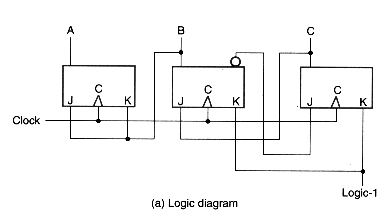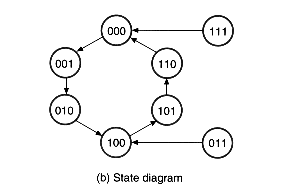### registers and counters

The ideas in combinational circuits (discussed in topics 2-4) and sequential methods (discussed in topics 5 and 6) when brought together as one system gives sequential building blocks, usually in the form or registers and counters.

A register is a set of flip-flops with combinational logic to implement state transitions that allow information to be stored and retrieved from them.  In the simplest form, a flip-flop is a one-bit register.

A counter is simply a register with combinational logic to implement counting, that is it is possible to retrieve the contents, add or subtract one to the contents, and then store it back into the register in one operation.

### four-bit register

A simple four-bit register with combinational logic to allow storage and retrieval is illustrated below.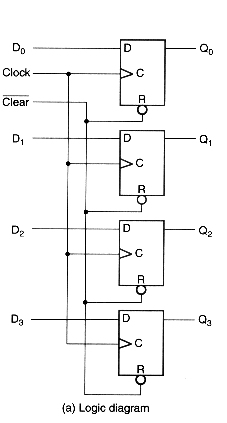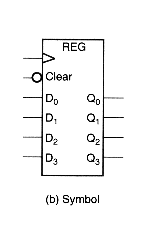The clear input is asynchronous and immediately clears the contents to zeroes.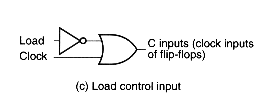Because the D-type flip-flop does not have a hold mode and will always cause the copying of its D inputs to the Q outputs on every clock pulse, we gate the clock (not nice) to allow conditional load of the register.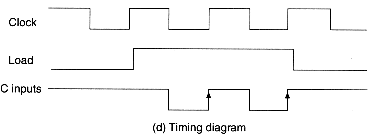The resultant behaviour is as expected, as illustrated by the timing diagram above.

Here we implement a synchronous counter in which the load function is synchronised with the clock, and the register is capable of hold mode even with when a clock edge occurs.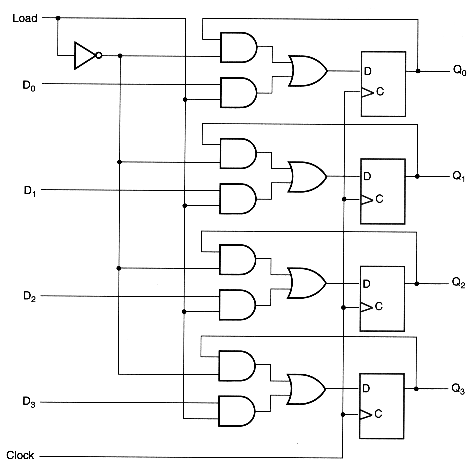Note that we use a 2-1 multiplexer at the inputs to either copy its current contents, or the new data from inputs, and make this selection based on the load signal.

### serial transfer

The cost of implementation of a parallel combination circuit can be reduced by using sequential circuits and with one (instead of multiple) functional component (that we consider expensive) as illustrated below.

First a register that allows contents to be shifted in and out serially.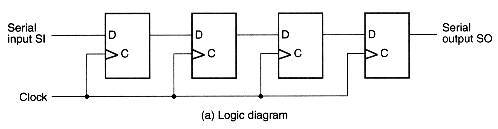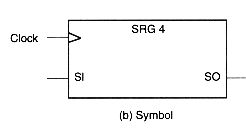We can transfer contents of one 4-bit register to another 4-bit register using only one data line as follows.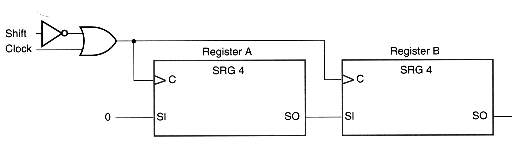Here we put a one-bit full adder with a carry flip-flop to implement a 4-bit serial full adder.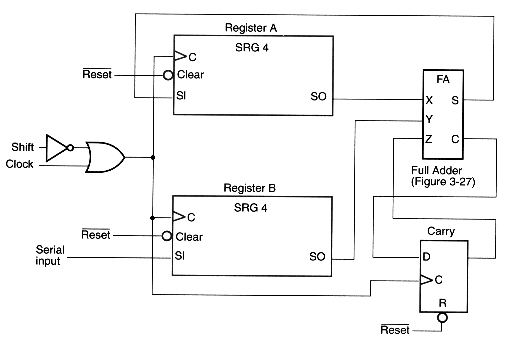The contents of register A are replaced by the sum of the contents of registers A and B.  What about register B?

### universal shift register

It is useful sequential building block allows serial shifts and parallel load functions.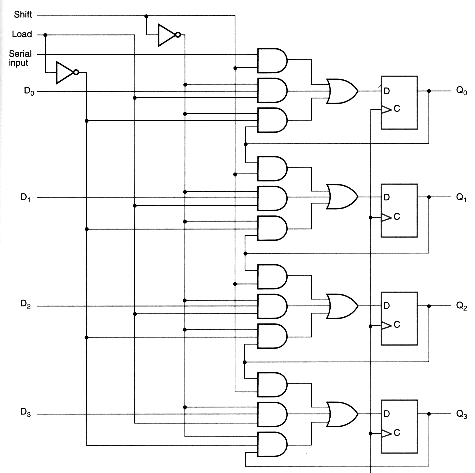We can simply the design using combination building blocks, and the example below, called a universal shift register allows serial shift in either direction.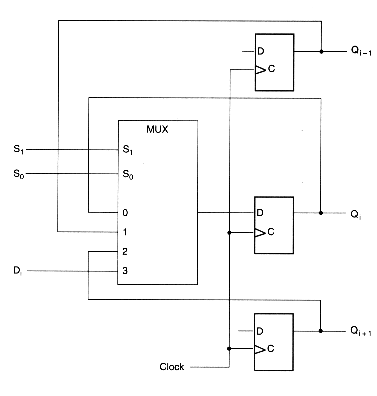### counters

A four-bit up-counter using J-K flip-flops is shown below.  This will always count on each clock pulse.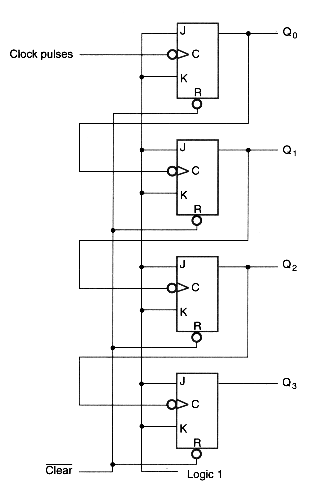When we need to count based on a given enable signal, the ripple carry problem emerges.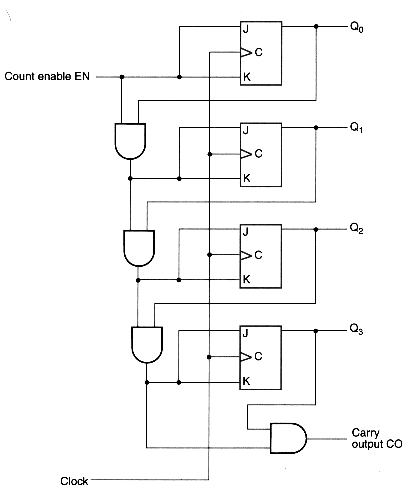In the case of an implementation using D-type flip-flops, we can use serial gating to propagate the enable signal as shown below.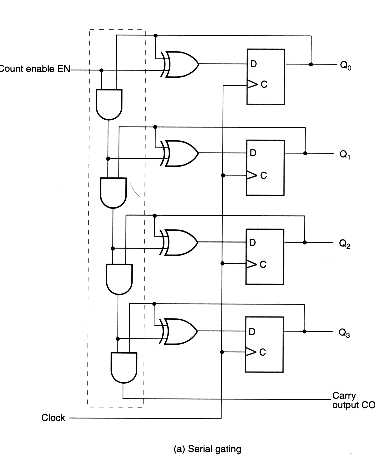Alternatively a faster parallel gating is shown but this involves gating the clock signal itself.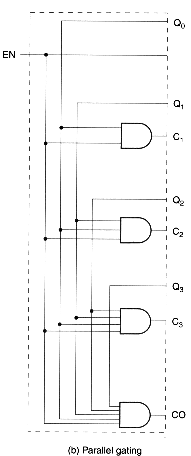### arbitrary counter

The following is an example of a counter which counts through an arbitrary sequence.